## AVERAGE Function

The AVERAGE function is a premade function in Google Sheets, which calculates the average (arithmetic mean).

It is typed `=AVERAGE` and gets a list of cells:

=AVERAGE(value1, [value2, ...])

It adds up all the cell values and divides that sum by the total number of values.

Example:

The average of the values (2, 3, 4) is 3.
There are 3 values in total
The sum of the values is (2 + 3 + 4 = 9)
(9 / 3 = 3)
The average is 3

Note: There are multiple types of mean values. The most common type of mean is the arithmetic mean. You can learn more about the arithmetic mean here: Statistics Mean Chapter.

Note: The AVERAGE function ignores cells with text.

## Average Function Example

Let's have a look at an example where we help the Pokemon trainers to calculate the average of Pokeballs per trainer.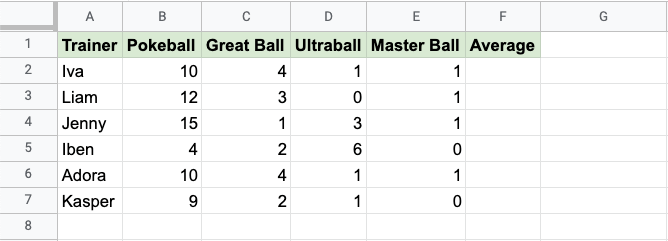Copy Values

How to use the `=AVERAGE` function, step by step:

1. Select the cell `F2`
2. Type `=AVERAGE`
3. Click the AVERAGE command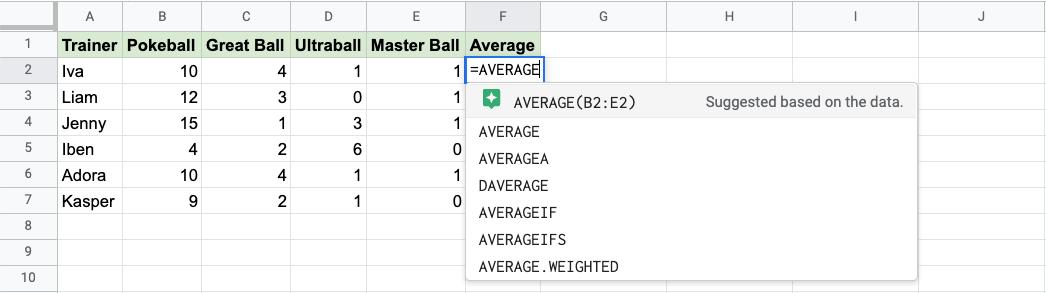Google Sheets will often give suggestions of how to complete your function based on the data in the spreadsheet.

In this case, the suggestion is precisely what we are looking for, the range `B2:E2`.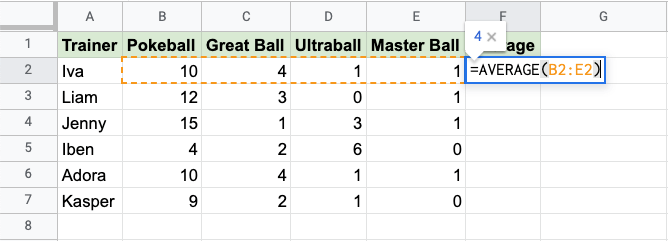Click the suggestion, hit enter, and the function is complete!

The function can be repeated with the filling function for each row to calculate the average number of Pokeballs for all the Trainers: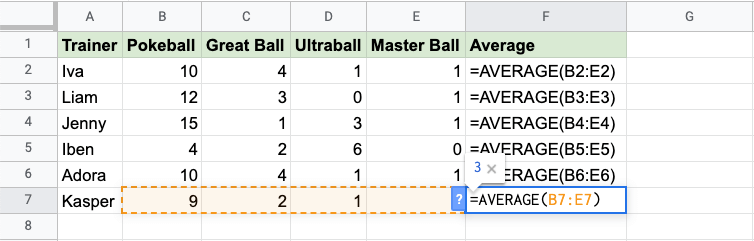Good job! You have successfully calculated the average using the AVERAGE function.

## Another Example

The suggestions are often very helpful for doing things quickly, but don't always suggest precisely what we want.

Let's have a look at a similar example, where the Pokeon trainers have another item in their inventory: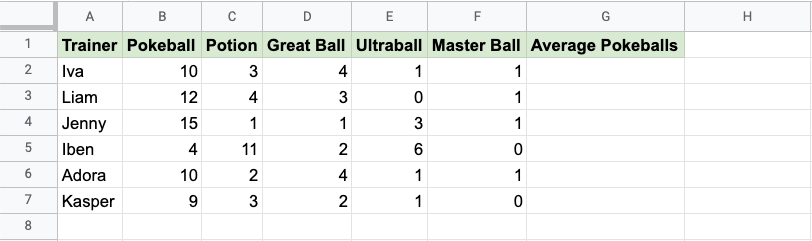Copy Values

How to use the `=AVERAGE` function, step by step:

1. Select the cell `E2`
2. Type `=AVERAGE`
3. Click the AVERAGE command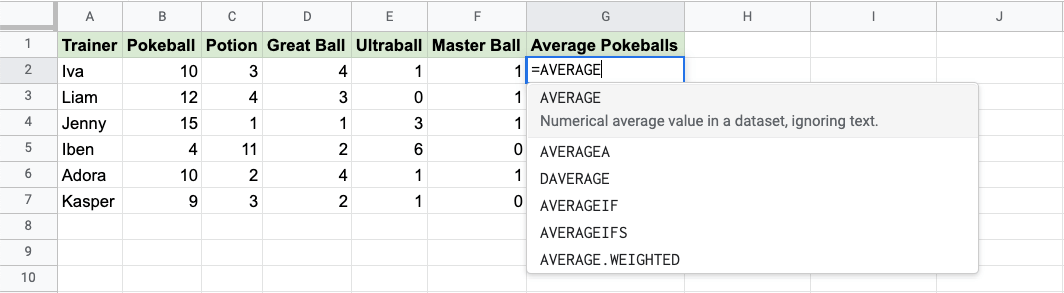1. Specify the cell `B2` for the Pokeballs
2. Type `,`
3. Specify the range `D2:F2` for the rest of the Pokeballs
4. Hit enter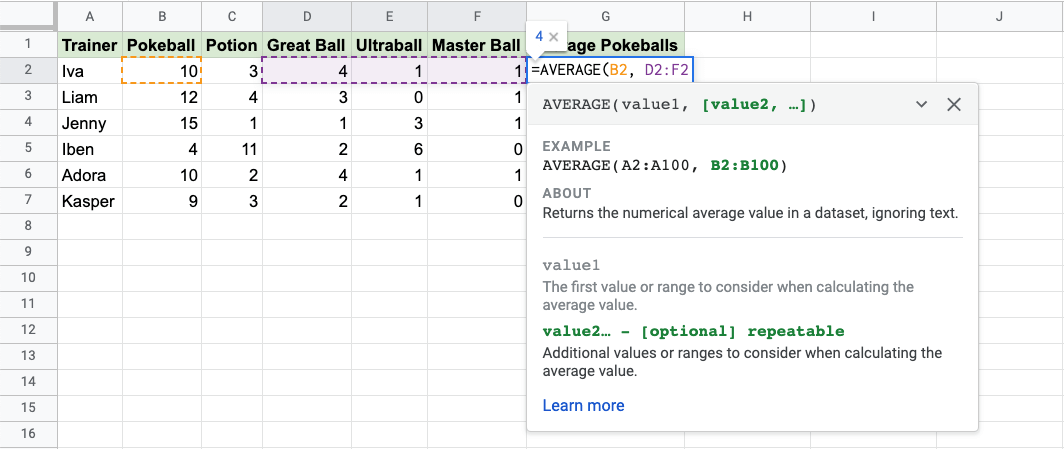The function now calculates the average of the cell `B2` together with the range `D2:F2`.

The function can be repeated with the filling function for each row to calculate the average number of Pokeballs for all the Trainers:Now, we can see the average number of Pokeballs for each of the trainers:W3Schools is optimized for learning and training. Examples might be simplified to improve reading and learning. Tutorials, references, and examples are constantly reviewed to avoid errors, but we cannot warrant full correctness of all content. While using W3Schools, you agree to have read and accepted our terms of use, cookie and privacy policy.